# RS Aggarwal Solutions for Class 7 Maths Exercise 18A Chapter 18 Reflection and Rotational Symmetry

RS Aggarwal Solutions for Class 7 Maths Exercise 18A Chapter 18 Reflection and Rotational Symmetry are given here in a simple and detailed way. Topic covered in this exercise is reflection symmetry, a figure is said to be symmetrical about a line ‘l’, if it is identical on either side of ‘l’. In this case, ‘l’ is called the line of symmetry. The RS Aggarwal Solutions for Class 7 Maths Chapter 18 can be extremely helpful for the students to clear all their doubts, and understand the basics of this chapter in a comprehensive manner. Access free PDF of RS Aggarwal Solutions for Class 7 Maths Chapter 18 Reflection and Rotational Symmetry, is available here.

## Download the PDF of RS Aggarwal Solutions For Class 7 Maths Chapter 18 Reflection and Rotational Symmetry – Exercise 18A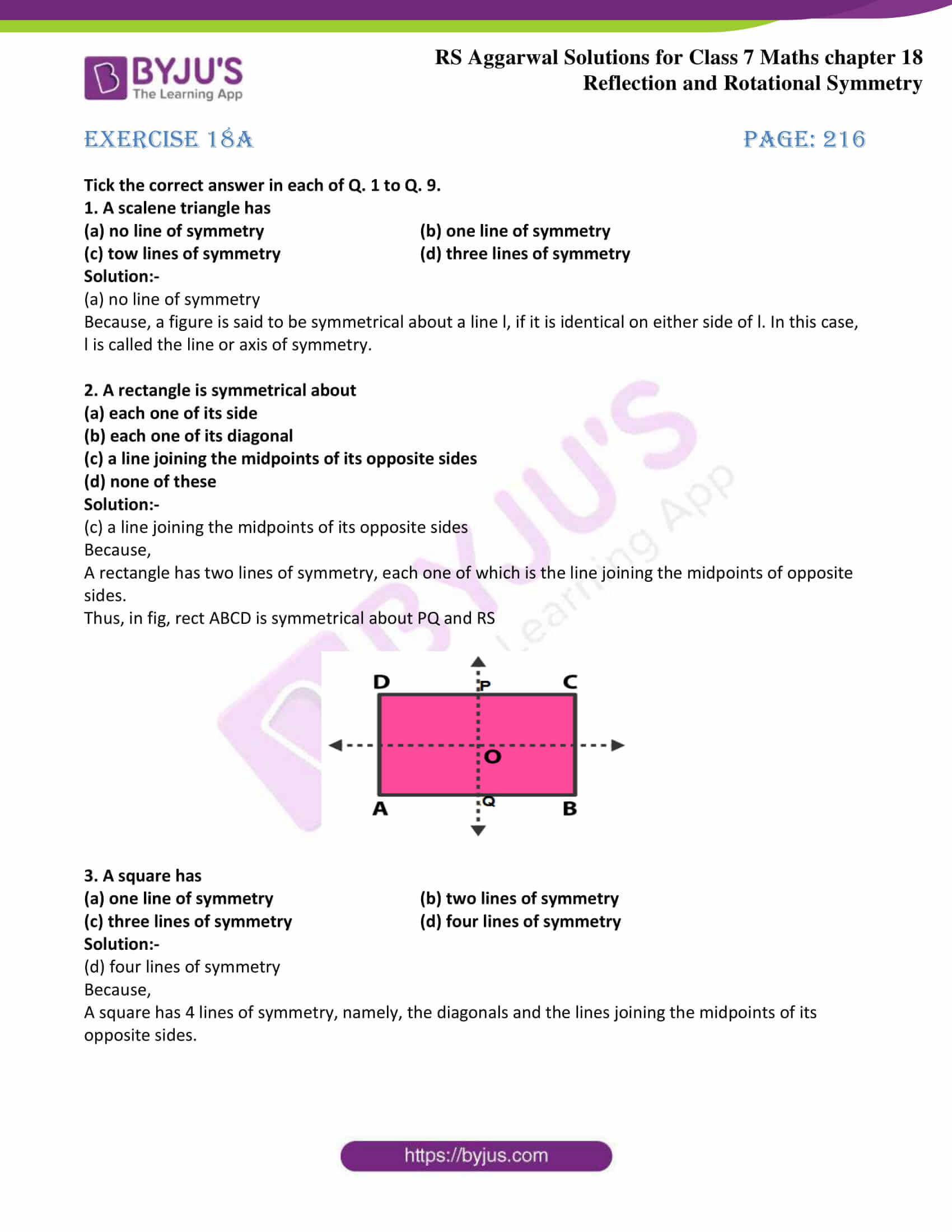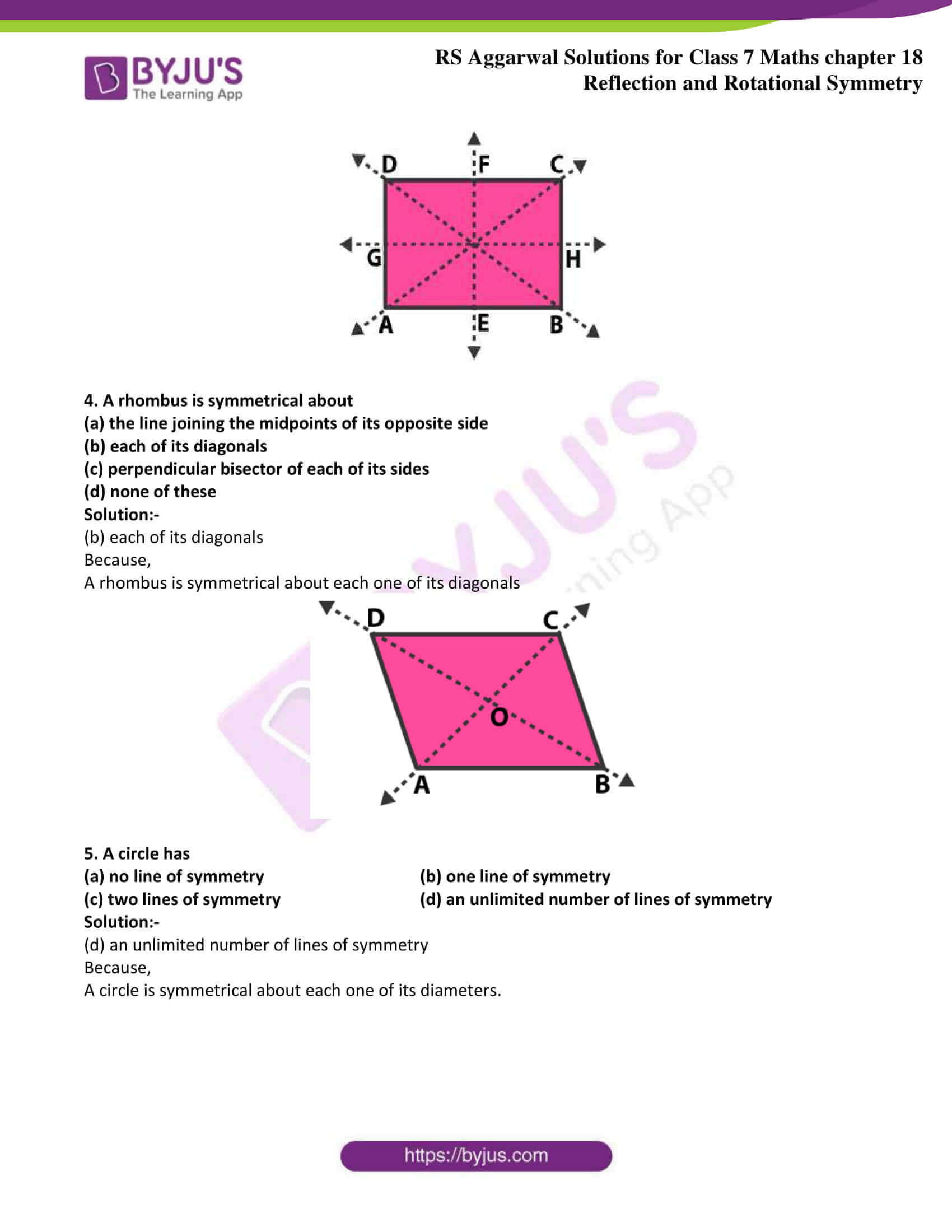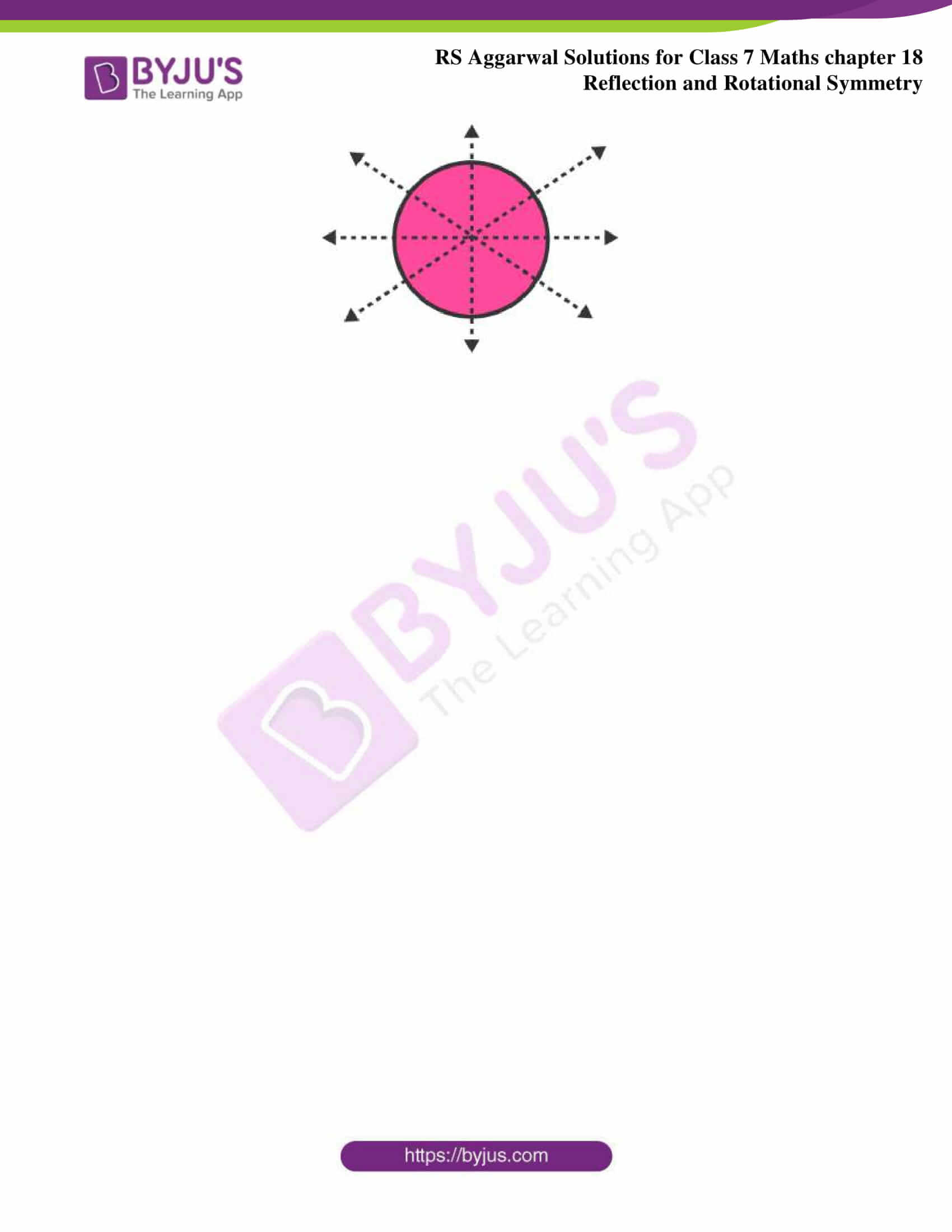### Access answers to Maths RS Aggarwal Solutions for Class 7 Chapter 18 – Reflection and Rotational Symmetry Exercise 18A

Tick the correct answer in each of Q. 1 to Q. 9.

1. A scalene triangle has

(a) no line of symmetry (b) one line of symmetry

(c) tow lines of symmetry (d) three lines of symmetry

Solution:-

(a) no line of symmetry

Because, a figure is said to be symmetrical about a line l, if it is identical on either side of l. In this case, l is called the line or axis of symmetry.

2. A rectangle is symmetrical about

(a) each one of its side

(b) each one of its diagonal

(c) a line joining the midpoints of its opposite sides

(d) none of these

Solution:-

(c) a line joining the midpoints of its opposite sides

Because,

A rectangle has two lines of symmetry, each one of which is the line joining the midpoints of opposite sides.

Thus, in fig, rect ABCD is symmetrical about PQ and RS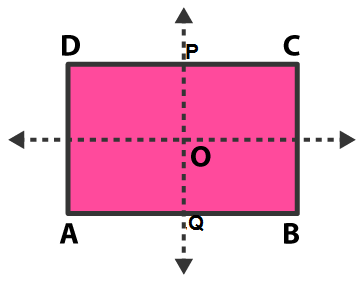3. A square has

(a) one line of symmetry (b) two lines of symmetry

(c) three lines of symmetry (d) four lines of symmetry

Solution:-

(d) four lines of symmetry

Because,

A square has 4 lines of symmetry, namely, the diagonals and the lines joining the midpoints of its opposite sides.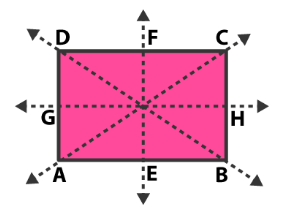4. A rhombus is symmetrical about

(a) the line joining the midpoints of its opposite side

(b) each of its diagonals

(c) perpendicular bisector of each of its sides

(d) none of these

Solution:-

(b) each of its diagonals

Because,

A rhombus is symmetrical about each one of its diagonals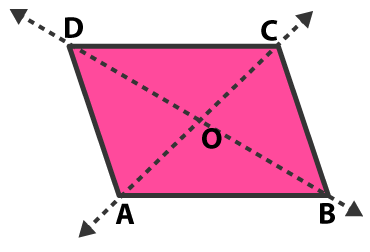5. A circle has

(a) no line of symmetry (b) one line of symmetry

(c) two lines of symmetry (d) an unlimited number of lines of symmetry

Solution:-

(d) an unlimited number of lines of symmetry

Because,

A circle is symmetrical about each one of its diameters.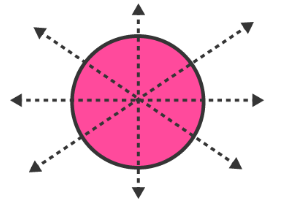### Access other exercises of RS Aggarwal Solutions For Class 7 Chapter 18 – Reflection and Rotational Symmetry

Exercise 18B Solutions